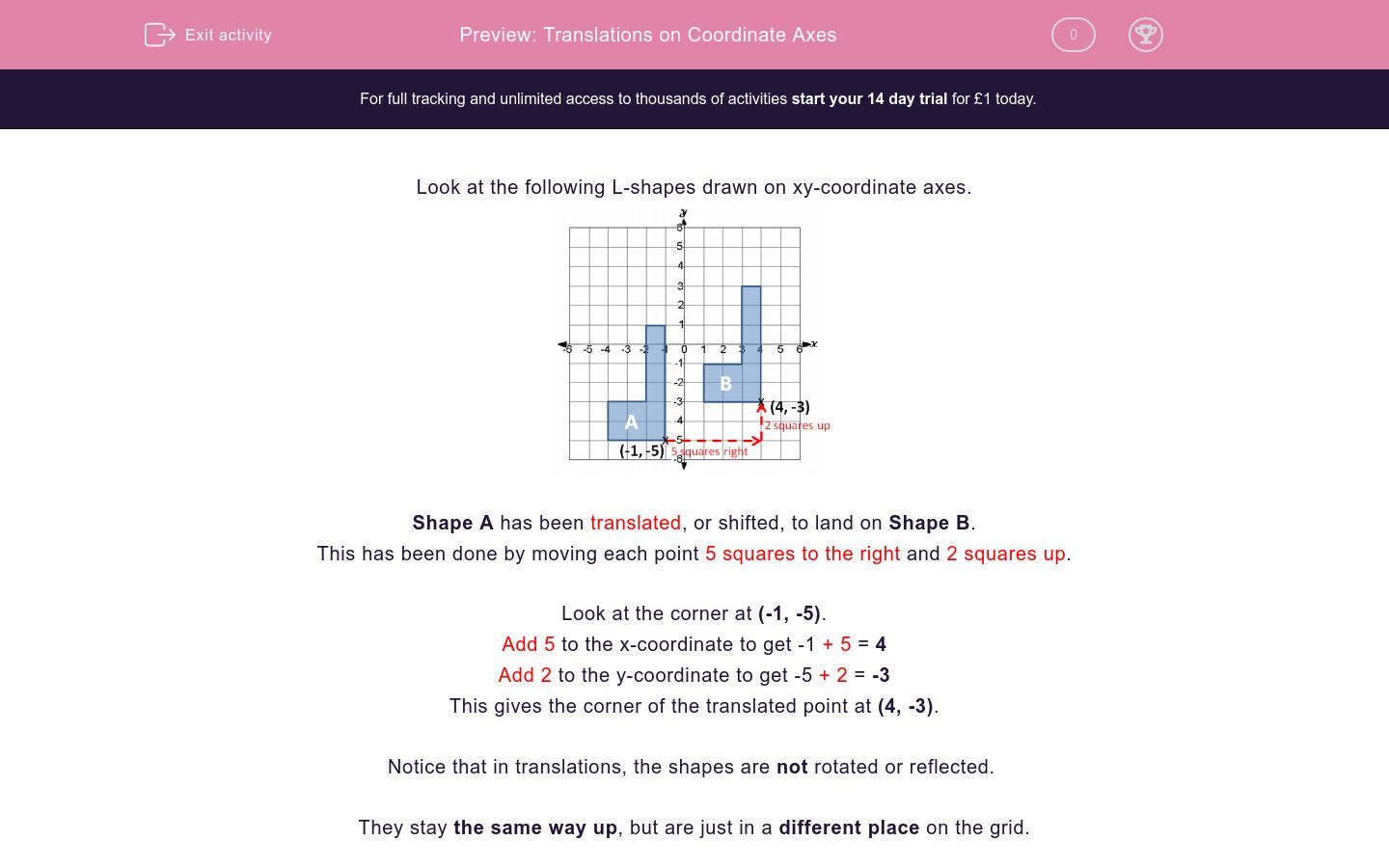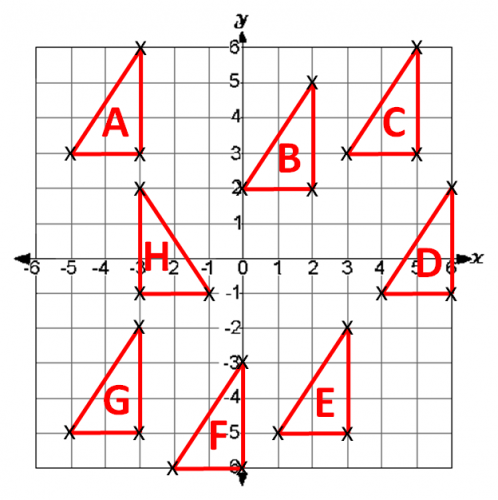# Translations on Coordinate Axes

In this worksheet, students translate shapes on a coordinate grid.Key stage:  KS 2

Curriculum topic:   Maths and Numerical Reasoning

Curriculum subtopic:   Transformations

Difficulty level:#### Worksheet Overview

Look at the following L-shapes drawn on xy-coordinate axes.Shape A has been translated, or shifted, to land on Shape B.

This has been done by moving each point 5 squares to the right and 2 squares up.

Look at the corner at (-1, -5).

Add 5 to the x-coordinate to get -1 + 5 = 4

Add 2 to the y-coordinate to get -5 + 2 = -3

This gives the corner of the translated point at (4, -3).

Notice that in translations, the shapes are not rotated or reflected.

They stay the same way up, but are just in a different place on the grid.

Example

Look at the triangles on this coordinate grid.On which triangle does Triangle B land when it is translated:

3 squares to the right and 1 square up